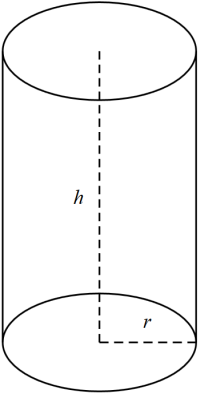# Cylinder Volume and Area Calculator

This calculator allows you to calculate the volume and area of a cylinder when we know the height of the cylinder and the area of its base or the radius of its base circle.

## Volume and Area of the Cylinder

The volume `V` of a right cylinder is calculated using the formula:

`V = Ah`, where `A` is the area of the cylinder's base and `h` is the height.

if the radius of the base circle is known:

`V = pir^2h`, where `r` is the radius of the base circle and `h` is the height.